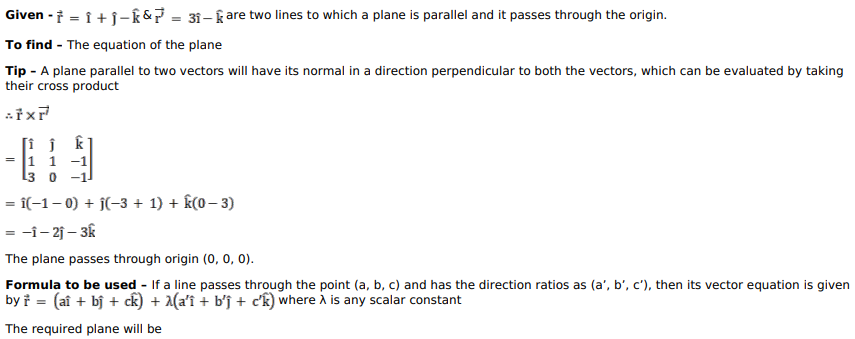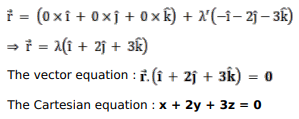# Find the vector and Cartesian equations

Question:

Find the vector and Cartesian equations of the plane passing through the origin and parallel to the vectors $(\hat{\mathrm{i}}+\hat{\mathrm{j}}-\hat{\mathrm{k}})$ and $(3 \hat{\mathrm{i}}-\hat{\mathrm{k}})$.

Solution: